Home > MC2 > Chapter 11 > Lesson 11.2.2 > Problem11-43

11-43.
1. Solve the following equations. Homework Help ✎

1.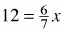2. 3x + (–4) = 2x + 9

3. 3x – 8 = 4x + (–2)

4. 2x – 6 = 1 – 3x

5.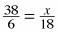6.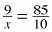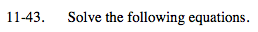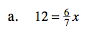Isolate the variable.

$\text{Multiply both sides of the equation by \frac{7}{6}}$

$\left( \frac{7}{6} \right) 12 = \frac{6}{7} x \left( \frac{7}{6} \right)$

$x = \frac{7}{6} (12) = 14$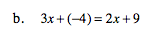3x + (−4) = 2x + 9

x + (−4) = 9

x = 13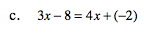See (b).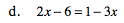See (b).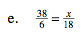You can multiply both sides by 18.

$\left( \frac{38}{6} \right) 18 = \left( \frac{x}{18} \right)18$

$x = \frac{684}{6} = 114$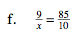See (e).

$x = \frac{90}{85}$